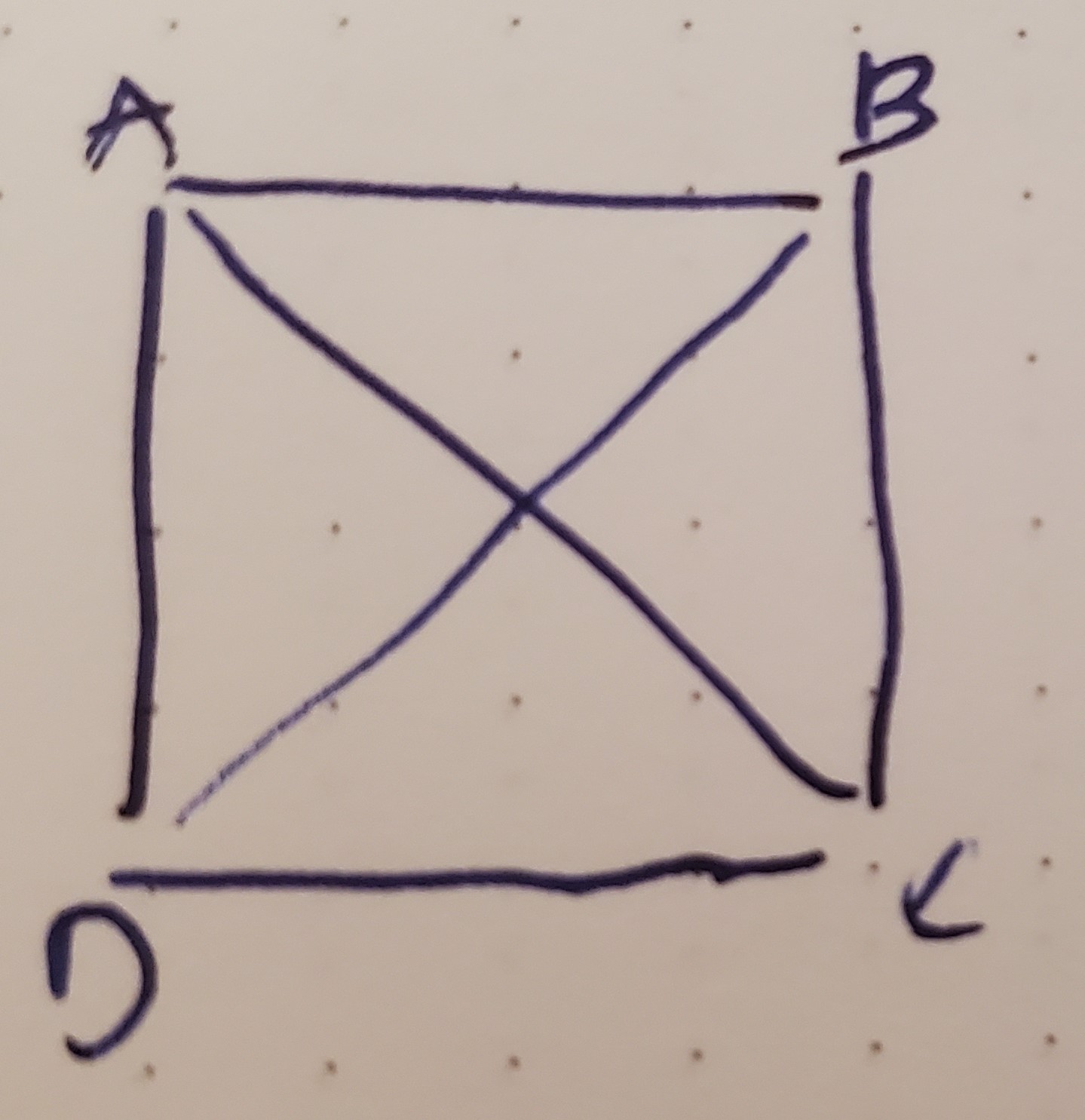### TASK #1 › Ugly Numbers

You are given an integer \$n >= 1.

Write a script to find the \$nth element of Ugly Numbers.

Ugly numbers are those number whose prime factors are 2, 3 or 5. For example, the first 10 Ugly Numbers are 1, 2, 3, 4, 5, 6, 8, 9, 10, 12.

ETA: I got this wrong. Corrected in another blog post.

I thought about Recursion, because I know my brand, but really, no. This is perfectly doable in an iterative way. I do it with an infinite while, but I could’ve done `for ( my \$i = 1; \$i <= \$n ; ) { ... }` and iterate within the loop only when we find another Ugly Number, or something like that.

#### Show Me The Code

``````#!/usr/bin/env perl

use feature qw{say state signatures};
use strict;
use warnings;
use utf8;
no warnings qw{ experimental };

use Carp;
use Getopt::Long;

my \$n = 8;

GetOptions( 'n=i' => \\$n, );
carp 'Bad Input' unless \$n > 0;

my \$u = get_ugly(\$n);
say "Input:  \\$n = \$n";
say "Output: \$u";

sub get_ugly ( \$n ) {
return 1 if \$n == 1;
my \$c = 1;
my \$u = 0;
while (1) {
\$u++;
my \$f = 0;
\$f = 1 if \$u % 2 == 0;
\$f = 1 if \$u % 3 == 0;
\$f = 1 if \$u % 5 == 0;
\$c++      if \$f;
return \$u if \$n == \$c;
}
}
``````
``````\$ time ./ch-1.pl -n 2000067
Input:  \$n = 2000067
Output: 2727363

real    0m0.645s
user    0m0.563s
sys     0m0.047s
\$ time ./ch-1.pl -n 20000678
Input:  \$n = 20000678
Output: 27273651

real    0m6.482s
user    0m6.328s
sys     0m0.016s
\$ time ./ch-1.pl -n 200006789
Input:  \$n = 200006789
Output: 272736530

real    1m11.287s
user    1m9.078s
sys     0m0.297s
``````

### TASK #2 › Square Points

You are given coordinates of four points i.e. (x1, y1), (x2, y2), (x3, y3) and (x4, y4).

Write a script to find out if the given four points form a square.

How are squares defined? Four sides of equal length and four corners with equal angles. If it has equal angles but not equal sides, it’s a rectangle. If it has equal sides but not equal angles, it’s a parallelogram. So, given points `A`, `B`, `C`, and `D`, you need to compare the distances `A->B`, `B->C`, `C->D` and `D->A`. But you also need to check the angles `ABC`, `BCD`, `CDA` and `DAC`.Or do you?

Because if `ABCD` is a parallelogram, `A->C` will be wildly different from `B->D`, while for a square, they will be equal.

Plus, you only have to remember one formula from high school math, c2 = a2 + b2, which we change to c = √a2 + b2, or c = √ (abs(x1 - x2 ))2 + (abs(y1 - y2 ))2

(I first wrote it with abs, but since a negative number multiplied by a negative number is a positive number, this should be unnecessary. Ah well. I do have some belt-and-suspenders tendencies…)

Speaking of, I wrote a graphing function to show me the whole, but removed it, because I was starting to think through parallelograms and it wouldn’t have handled floating-point locations. Maybe a HTML/JS/CSS/SVG version would be the way to show the points and shape, as well as discerning if it’s square or not. Looks like I’ll want to make a second solution and blog post.

#### Show Me The Code

``````#!/usr/bin/env perl

use feature qw{say state signatures};
use strict;
use warnings;
use utf8;
no warnings qw{ experimental };

use JSON;

my \$json = JSON->new->canonical;
my @data = (
[ [ 10, 20 ], [ 20, 20 ], [ 20, 10 ], [ 10, 10 ], ],
[ [ 12, 24 ], [ 16, 10 ], [ 20, 12 ], [ 18, 16 ], ],
[ [ 40, 40 ], [ 50, 30 ], [ 40, 20 ], [ 30, 30 ], ],
[ [ 10, 10 ], [ 15, 15 ], [ 20, 15 ], [ 15, 10 ], ],
);

for my \$d (@data) {
say \$json->encode(\$d);
say is_square(\$d);
say '';
}

sub is_square(\$d) {
my @objs = \$d->@*;
my @distances;
push @distances, distance( @objs[ 0, 1 ] ); # A -> B
push @distances, distance( @objs[ 1, 2 ] ); # B -> C
push @distances, distance( @objs[ 2, 3 ] ); # C -> D
push @distances, distance( @objs[ 3, 0 ] ); # D -> A

push @distances, distance( @objs[ 0, 2 ] ); # A -> C
push @distances, distance( @objs[ 1, 3 ] ); # B -> D

# sides are of equal length
return 0 if \$distances != \$distances;
return 0 if \$distances != \$distances;
return 0 if \$distances != \$distances;
return 0 if \$distances != \$distances;

# distances throught the center are of equal length
# removing parallelograms
return 0 if \$distances != \$distances;

return 1;
}

sub distance ( \$p1, \$p2 ) {
return
sqrt( ( ( \$p1-> - \$p2-> )**2 ) +
( ( \$p1-> - \$p2-> )**2 ) );
}
``````
``````
[[10,20],[20,20],[20,10],[10,10]]
1

[[12,24],[16,10],[20,12],[18,16]]
0

[[40,40],[50,30],[40,20],[30,30]]
1

[[10,10],[15,15],[20,15],[15,10]]
0
``````